# ThermalConductor

Lumped thermal element transporting heat without storing it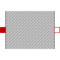# Information

This information is part of the Modelica Standard Library maintained by the Modelica Association.

This is a model for transport of heat without storing it; see also: ThermalResistor. It may be used for complicated geometries where the thermal conductance G (= inverse of thermal resistance) is determined by measurements and is assumed to be constant over the range of operations. If the component consists mainly of one type of material and a regular geometry, it may be calculated, e.g., with one of the following equations:

• Conductance for a box geometry under the assumption that heat flows along the box length:

```    G = k*A/L
k: Thermal conductivity (material constant)
A: Area of box
L: Length of box
```
• Conductance for a cylindrical geometry under the assumption that heat flows from the inside to the outside radius of the cylinder:

```    G = 2*pi*k*L/log(r_out/r_in)
pi   : Modelica.Constants.pi
k    : Thermal conductivity (material constant)
L    : Length of cylinder
log  : Modelica.Math.log;
r_in : Inner radius of cylinder
```
```    Typical values for k at 20 degC in W/(m.K):
aluminium   220
concrete      1
copper      384
iron         74
silver      407
steel        45 .. 15 (V2A)
wood         0.1 ... 0.2
```

# Parameters (1)

G Value: Type: ThermalConductance (W/K) Description: Constant thermal conductance of material

# Connectors (2)

port_a port_b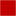Type: HeatPort_a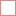Type: HeatPort_b

# Used in Examples (13)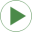HeatingMOSInverter Modelica.Electrical.Analog.Examples Heating MOS InverterHeatingNPN_OrGate Modelica.Electrical.Analog.Examples Heating NPN Or GateHeatingPNP_NORGate Modelica.Electrical.Analog.Examples Heating PNP NOR GateHeatingResistor Modelica.Electrical.Analog.Examples Heating resistorHeatingRectifier Modelica.Electrical.Analog.Examples Heating rectifierDCPM_Cooling Modelica.Electrical.Machines.Examples.DCMachines Test example: Cooling of a DCPM motorHeatingSystem Modelica.Fluid.Examples Simple model of a heating systemIndirectCooling Modelica.Thermal.FluidHeatFlow.Examples Indirect cooling circuitOneMass Modelica.Thermal.FluidHeatFlow.Examples Cooling of one hot massTwoMass Modelica.Thermal.FluidHeatFlow.Examples Cooling of two hot massesTwoMasses Modelica.Thermal.HeatTransfer.Examples Simple conduction demoControlledTemperature Modelica.Thermal.HeatTransfer.Examples Control temperature of a resistorMotor Modelica.Thermal.HeatTransfer.Examples Second order thermal model of a motor

# Used in Components (1)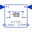Conduction Modelica.Thermal.HeatTransfer.Examples.Utilities Input/output block of a conduction model